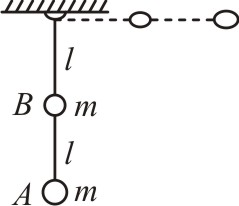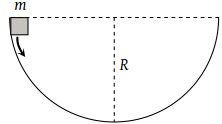# A weightless rod of length 2l carries two equal mass 'm', one tied at lower end A and the other at the middle of the rod at B. The rod can rotate in a vertical plane about a fixed horizontal axis passing through C. The rod is released from rest in the horizontal position. The speed of the mass B at the instant rod becomes vertical is:(1) $\sqrt{\frac{3gl}{5}}$ (2) $\sqrt{\frac{4gl}{5}}$ (3) $\sqrt{\frac{6gl}{5}}$ (4) $\sqrt{\frac{7gl}{5}}$

Subtopic:  Work Energy Theorem |
To view explanation, please take trial in the course below.
NEET 2023 - Target Batch - Aryan Raj Singh
To view explanation, please take trial in the course below.
NEET 2023 - Target Batch - Aryan Raj Singh
Launched MCQ Practice Books

Prefer Books for Question Practice? Get NEETprep's Unique MCQ Books with Online Audio/Video/Text Solutions via Telegram Bot

A force F is applied on a body which moves with a velocity v in the direction of the force, then the power will be

1.  ${\mathrm{Fv}}^{2}$

2.  Fv

3.  $\mathrm{F}/{\mathrm{v}}^{2}$

4.  F/v

Subtopic:  Power |
To view explanation, please take trial in the course below.
NEET 2023 - Target Batch - Aryan Raj Singh
To view explanation, please take trial in the course below.
NEET 2023 - Target Batch - Aryan Raj Singh
Launched MCQ Practice Books

Prefer Books for Question Practice? Get NEETprep's Unique MCQ Books with Online Audio/Video/Text Solutions via Telegram Bot

Potential energy (U) related to coordinates is given by U = 3(x + y). Work done by the conservative force when the particle is going from (0, 0), (2, 3) is:
1.  15 J
2.  -15 J
3.  12 J
4.  10 J

Subtopic:  Potential Energy: Relation with Force |
To view explanation, please take trial in the course below.
NEET 2023 - Target Batch - Aryan Raj Singh
To view explanation, please take trial in the course below.
NEET 2023 - Target Batch - Aryan Raj Singh
Launched MCQ Practice Books

Prefer Books for Question Practice? Get NEETprep's Unique MCQ Books with Online Audio/Video/Text Solutions via Telegram Bot

A mass m slips along the wall of a semispherical surface of radius R. The velocity at the bottom of the surface is [ MP PMT 1993](1) $\sqrt{Rg}$

(2) $\sqrt{2Rg}$

(3) $2\sqrt{\pi Rg}$

(4) $\sqrt{\pi Rg}$

Subtopic:  Gravitational Potential Energy |
To view explanation, please take trial in the course below.
NEET 2023 - Target Batch - Aryan Raj Singh
To view explanation, please take trial in the course below.
NEET 2023 - Target Batch - Aryan Raj Singh
Launched MCQ Practice Books

Prefer Books for Question Practice? Get NEETprep's Unique MCQ Books with Online Audio/Video/Text Solutions via Telegram Bot

Three different objects of mass  and m3 are allowed to fall from rest and from the same point ‘O’ along three different frictionless paths. The speeds of the three objects, on reaching the ground, will be in the ratio of:

1. ${m}_{1}:{m}_{2}:{m}_{3}$

2. ${m}_{1}:2{m}_{2}:3{m}_{3}$

3. 1 : 1 : 1

4. $\frac{1}{{m}_{1}}:\frac{1}{{m}_{2}}:\frac{1}{{m}_{3}}$

Subtopic:  Work Energy Theorem |
To view explanation, please take trial in the course below.
NEET 2023 - Target Batch - Aryan Raj Singh
To view explanation, please take trial in the course below.
NEET 2023 - Target Batch - Aryan Raj Singh
Launched MCQ Practice Books

Prefer Books for Question Practice? Get NEETprep's Unique MCQ Books with Online Audio/Video/Text Solutions via Telegram Bot

When a body moves with a constant speed along a circle

(1) No work is done on it

(2) No acceleration is produced in the body

(3) No force acts on the body

(4) Its velocity remains constant

Subtopic:  Work Done by Variable Force |
To view explanation, please take trial in the course below.
NEET 2023 - Target Batch - Aryan Raj Singh
To view explanation, please take trial in the course below.
NEET 2023 - Target Batch - Aryan Raj Singh
Launched MCQ Practice Books

Prefer Books for Question Practice? Get NEETprep's Unique MCQ Books with Online Audio/Video/Text Solutions via Telegram Bot

A sphere of mass m is tied to end of a string of length l and rotated through the other end along a horizontal circular path with speed v. The work done by centripetal force in full horizontal circle is

(1) 0

(2) $\left(\frac{m{v}^{2}}{l}\right) . 2\pi l$

(3) $\text{mg\hspace{0.17em}.\hspace{0.17em}2πl}$

(4) $\left(\frac{m{v}^{2}}{l}\right) . \left(l\right)$

Subtopic:  Work Done by Variable Force |
To view explanation, please take trial in the course below.
NEET 2023 - Target Batch - Aryan Raj Singh
To view explanation, please take trial in the course below.
NEET 2023 - Target Batch - Aryan Raj Singh
Launched MCQ Practice Books

Prefer Books for Question Practice? Get NEETprep's Unique MCQ Books with Online Audio/Video/Text Solutions via Telegram Bot

In a circus stuntman rides a motorbike in a circular track of radius R in the vertical plane. The minimum speed at highest point of track will be

(1) $\sqrt{2gR}$

(2) 2gR

(3) $\sqrt{3gR}$

(4) $\sqrt{gR}$

Subtopic:  Work Energy Theorem |
To view explanation, please take trial in the course below.
NEET 2023 - Target Batch - Aryan Raj Singh
To view explanation, please take trial in the course below.
NEET 2023 - Target Batch - Aryan Raj Singh
Launched MCQ Practice Books

Prefer Books for Question Practice? Get NEETprep's Unique MCQ Books with Online Audio/Video/Text Solutions via Telegram Bot

A ball is suspended by a thread of length l. What minimum horizontal velocity has to be imparted to the ball for it to reach the height of the suspension:

(1) gl

(2) 2 gl

(3) $\sqrt{gl}$

(4) $\sqrt{2gl}$

Subtopic:  Work Energy Theorem |
To view explanation, please take trial in the course below.
NEET 2023 - Target Batch - Aryan Raj Singh
To view explanation, please take trial in the course below.
NEET 2023 - Target Batch - Aryan Raj Singh
Launched MCQ Practice Books

Prefer Books for Question Practice? Get NEETprep's Unique MCQ Books with Online Audio/Video/Text Solutions via Telegram Bot

A body of mass m hangs at one end of a string of length l, the other end of which is fixed. It is given a horizontal velocity so that the string would just reach where it makes an angle of 60° with the vertical. The tension in the string at mean position is

(1) 2 mg

(2) mg

(3) 3 mg

(4) $\sqrt{3}mg$

Subtopic:  Work Energy Theorem |
To view explanation, please take trial in the course below.
NEET 2023 - Target Batch - Aryan Raj Singh
To view explanation, please take trial in the course below.
NEET 2023 - Target Batch - Aryan Raj Singh